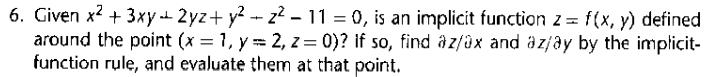Home / Expert Answers / Prealgebra / 6-given-x-2-3-x-y-2-y-z-y-2-z-2-11-0-is-an-implicit-function-pa767

# (Solved): 6. Given $$x^{2}+3 x y+2 y z+y^{2}-z^{2}-11=0$$, is an implicit function $$...??????? 6. Given \( x^{2}+3 x y+2 y z+y^{2}-z^{2}-11=0$$, is an implicit function $$z=f(x, y)$$ defined around the point $$(x=1, y=2, z=0)$$ ? If so, find $$\partial z / \partial x$$ and $$\partial z / \partial y$$ by the implicitfunction rule, and evaluate them at that point.

We have an Answer from Expert